### Confidence Intervals

By default, the SURVEYPHREG procedure computes t confidence limits for the estimated regression coefficients. Alternatively, you can specify the DF=NONE option in the MODEL statement to request standard normal confidence intervals. The t confidence interval for a linear combination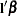of the regression coefficients is computed as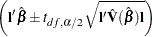where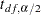is the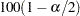percentile point of thedistribution with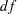degrees of freedom. See the section Degrees of Freedom for more information about. If you use the DF=NONE option in the MODEL statement, then the procedure uses thepercentile point of the standard normal distribution.